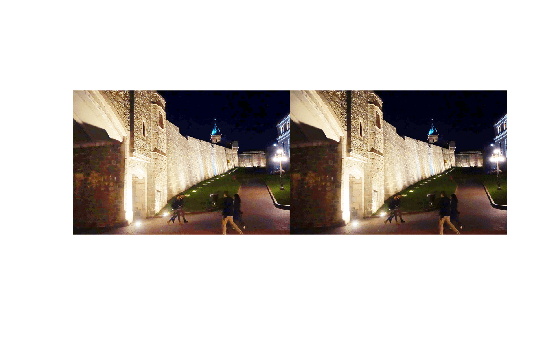Documentation

# imlocalbrighten

Brighten low-light image

## Syntax

``B = imlocalbrighten(A)``
``B = imlocalbrighten(A,amount)``
``[B,D] = imlocalbrighten(___)``
``[___] = imlocalbrighten(___,'AlphaBlend',TF)``

## Description

example

````B = imlocalbrighten(A)` brightens low-light areas in `A`, which is an RGB or grayscale image. Returns `B`, the locally brightened image, which is the same size and class as `A`.```
````B = imlocalbrighten(A,amount)` brightens low-light areas in `A`, where `amount` specifies how much to brighten the dark areas of the image. Specify `amount` as a scalar in the range `[0,1]`.```
````[B,D] = imlocalbrighten(___)` returns the optional argument `D`, which is a matrix containing estimates of how dark each pixel is in the input image.```
````[___] = imlocalbrighten(___,'AlphaBlend',TF)` brightens low-light areas in `A`, where `'AlphaBlend'` specifies whether you want to preserve content of the input image in the output image. Specify `TF` as `true` or `false` (default). When `true`, `imlocalbrighten` alpha blends the input image with the enhanced image to preserve brighter areas of the input image. `imlocalbrighten` uses the estimate of darkness matrix, `D`, to preserve content of the input image proportional to the amount of light in each pixel. The parameter name can be abbreviated, and case does not matter.```

## Examples

collapse all

Brighten a low-light image using default parameters. View the original image and the lightened version of the image.

```A = imread('lowlight_2.jpg'); B = imlocalbrighten(A); figure, imshowpair(A,B,'montage')```Brighten the low-light image again, this time specifying the amount of lightening to apply to the image. View the original image and this lightened version of the image.

```A = imread('lowlight_2.jpg'); B2 = imlocalbrighten(A,0.8); figure, imshowpair(A,B2,'montage')```Use the `AlphaBlend` option to preserve content from the original image in the lightened image. This time, view the lightened output image from the first example with the alpha blended output image. Compare the detail shown in the wall above arched entryway near the center of the image in the alpha-blended version with the original lightened image.

```A = imread('lowlight_2.jpg'); Bblend = imlocalbrighten(A,'AlphaBlend',true); figure, imshowpair(B,Bblend,'montage')```Get the estimated darkness-per-pixel matrix return value. View the original image and the darkness estimate matrix.

```A = imread('lowlight_2.jpg'); [~,D] = imlocalbrighten(A); figure, imshowpair(A,D,'montage')```## Input Arguments

collapse all

Image to be brightened, specified as a real, nonsparse, grayscale image (M-by-N) or RGB image (M-by-N-by-3).

Data Types: `single` | `double` | `uint8` | `uint16`

How much to brighten the image, specified as a numeric scalar in the range `[0 1]`. When the value is `1` (the default), `imlocalbrighten` brightens the low-light areas of `A` as much as possible. When the value is `0`, `imlocalbrighten` returns the input image unmodified.

Example: `0.2`

Data Types: `single` | `double` | `int8` | `int16` | `int32` | `int64` | `uint8` | `uint16` | `uint32` | `uint64`

## Output Arguments

collapse all

Brightened image, returned as a grayscale or an RGB image, the same size and class as the input image `A`.

Estimate of darkness of each pixel in the input image, returned as a numeric matrix. `D` is the same size as the first two dimensions of the input image and is of class `double`

 Dong, X., G. Wang, Y. Pang, W. Li, J. Wen, W. Meng, and Y. Lu. "Fast efficient algorithm for enhancement of low lighting video." Proceedings of IEEE® International Conference on Multimedia and Expo (ICME). 2011, pp. 1–6.

 He, Kaiming. "Single Image Haze Removal Using Dark Channel Prior." Thesis, The Chinese University of Hong Kong, 2011.

 Dubok Park; Hyungjo Park; David K. Han; Hanseok Ko "Single Image Dehazing with Image Entropy and Information Fidelity." ICIP, 2014.